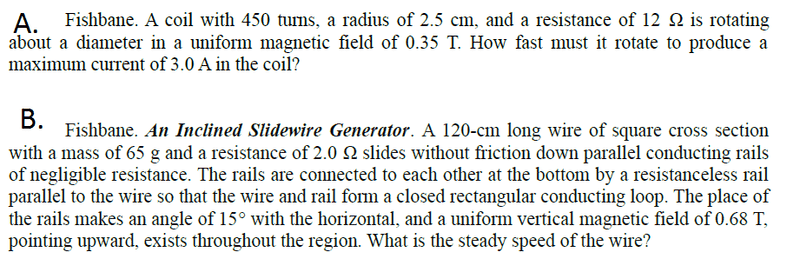# How fast must a coil be rotating to produce a certain current

## Homework Statement## The Attempt at a Solution

For letter A, my guess is ξ = dɸB/dt, ɸB = BA (since both magnetic field and the area are constants)

After substituting, I ended up with a hanging $\frac{d}{dt}$..

I'm quite confused.

For letter B, I don't know. Will I use the same formula I've used in letter A?

What result did you get? If you get a cos(wt) in your flux, you're on the right track.

What I have in hand is 116.504rad/s using the equation IR = wNBAsin(wt), with the assumption that the sin(wt) will become 1. (A=area, N=number of turns, w=angular speed)

I don't know how will I obtain t.

I don't know also if my answer is right..

Additional question, how can I resolve the forces in x and y component?

All I know is that Fx=m*a*cos(theta). I don't know for Fy.

You don't need t, the problem wants the maximum value. When is sine maximum?

I'm not sure what you mean for y without information on how you set up your axis. What do you need the force for anyway? Are you referring to do the second problem? The second problem is almost completely different from the first.

Yeah, I just used the equation Emax = wNBA, and Emax=ImaxR since when E is max, the sin(wt) should be equal to 1. And my answer is 19rev/s

For the second problem, I got around 1.9m/s. I don't know if I used the right equation. F=ILBsinx, F=mgsinx, E=-BLvcosx, E=IR, ending up with v=(mgR)/(B2L2cosx)